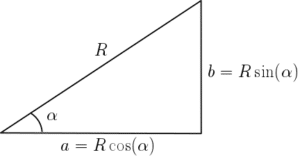# Compound Angle Identities – more Trigonometric Identities

## What are the Compound Angle Identities?

In addition to the basic trigonometric identities and the reciprocal identities there are the compound angle identities including the double angle identities. The compound angle identities (sometimes called the addition angle identities) are as follows:

$\begin{array}{l}\sin(A+B)\equiv \sin(A)\cos(B)+\cos(A)\sin(B)\\\cos(A+B)\equiv \cos(A)\cos(B)-\sin(A)\sin(B)\\\tan(A+B)\equiv\frac{\tan(A)+\tan(B)}{1-\tan(A)\tan(B)}\end{array}$

Proof of the first two identities follows from considering two compound triangles and proof of the third comes from using the first two identities (see video – coming soon). By replacing $B$ with $-B$ and noting that $\sin(-B)=-\sin(B)$, $\cos(-B)=\cos(B)$ and $\tan(-B)=-\tan(B)$ we also have:

$\begin{array}{l}\sin(A-B)\equiv \sin(A)\cos(B)-\cos(A)\sin(B)\\\cos(A-B)\equiv \cos(A)\cos(B)+\sin(A)\sin(B)\\\tan(A-B)\equiv\frac{\tan(A)-\tan(B)}{1+\tan(A)\tan(B)}\end{array}$

See Example 1 and videos for examples using the compound angle formulae.

## Double Angle Formulae

The double angle formulae (or identities) follow from the compound angle identities. By setting $B=A$ in the first set of identities above we obtain:

$\begin{array}{l}\sin(2A)\equiv 2\sin(A)\cos(A)\\\cos(2A)\equiv \cos^2(A)-\sin^2(A)\equiv 2\cos^2(A)-1\equiv 1-2\sin^2(A)\\\tan(2A)\equiv\frac{2\tan(A)}{1-\tan^2(A)}\end{array}$

Note that there are two additional identities for $\cos(2A)$ here. These come from using $\sin^2(A)+\cos^2(A)=1$ – see more trigonometric identities. It is worth memorising the double angle identities as it can save time when answering exam questions. See Example 2.

## Expressions of the form $a\sin(x)+b\cos(x)$

We can use the compound angle identities above to solve equations that are given in the form $a\sin(x)+b\cos(x)=c$, for example. We do this by converting the trigonometric expression into the form $R\sin(x+\alpha)$, for example. Using the identity $\sin(A+B)\equiv\sin(A)\cos(B)+\cos(A)\sin(B)$, we can write:

$R\sin(x+\alpha)\equiv R\sin(x)\cos(\alpha)+R\cos(x)\sin(\alpha)$

This expression now has separate $\sin(x)$ and $\cos(x)$ terms, the coefficients of which are $R\cos(\alpha)$ and $R\sin(\alpha)$. Comparing this with the expression $a\sin(x)+b\cos(x)$ we must equate the coefficients and so:

$\begin{array}{l}a&=&R\cos(\alpha)\\b&=&R\sin(\alpha)\end{array}$You might recognise these expressions from the right-angled triangle with hypoteneuse $R$ and angle $\alpha$. The sides can be found using SOHCAHTOA (what is SOHCAHTOA?). The opposite is $R\sin(\alpha)$ (which is $a$) and the adjacent is $R\cos(\alpha)$ (which is $b$). It follows that expressions of the form $a\sin(x)+b\cos(x)$ can be converted to expressions of the form $R\sin(x+\alpha)$ by setting $R=\sqrt{a^2+b^2}$ and $\alpha=\tan^{-1}\left(\frac{b}{a}\right)$. See Example 3. Note that the above process can be applied to any of the compound angle identities.

## Examples using Compound Angle Identities

1. Given that $\sin(x)=\frac{5}{13}$ for obtuse $x$ and $\cos(y)=\frac{4}{5}$ for acute $y$, find the value of $\sin(x-y)$.
2. Show that $\cos(75^\circ)=\frac{\sqrt{6}-\sqrt{2}}{4}$.
1. Solve $4\sin(2x)=\sin(x)$ for $0\leq x<2\pi$
2. Given the parametric equations $x=2\cos(2\theta)$ and $y=1-3\sin(\theta)$, find the Cartesian equation. (See more on parametric equations).
1. Express $3\sin(x)+4\cos(x)$ in the form $R\sin(x+\alpha)$. Hence solve the equation $3\sin(x)+4\cos(x)=2$ on the interval $-\pi\leq x\leq \pi$
2. Write $\cos(x)+\sqrt{2}\sin(x)$ in the form $R\cos(x-\alpha)$. Hence, sketch the curve of $y=\cos(x)-\sqrt{2}\sin(x)$.